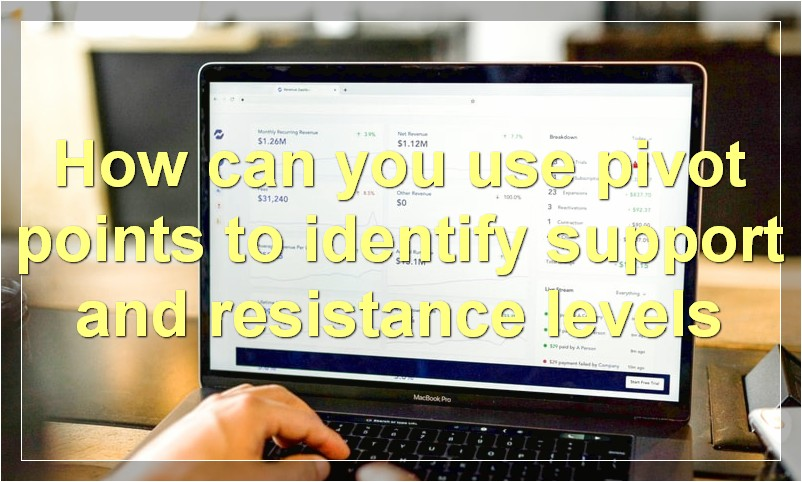# Pivot Points: Tips, Tricks, And Techniques

Pivot points are a great way to improve your trading skills. By following these simple tips, tricks, and techniques, you can make sure that you’re always making the most profitable trades possible.

## What are some tips for trading with pivot pointsPivot points are technical analysis tools that are used by traders to identify potential support and resistance levels in the market.

There are a few different ways to calculate pivot points, but the most common method is to take the average of the high, low, and close prices from the previous day. From there, traders can use these levels to help them make decision about where to enter or exit trades.

Here are a few tips for trading with pivot points:

1. Look for breakouts above or below key pivot levels.

2. Use pivot levels as targets for taking profits.

3. Use pivot levels to place stop-loss orders.

4. Watch for reversals at key pivot levels.

5. Be aware of false breakouts, which can often occur at pivot levels.

## How do you calculate pivot points

Pivot points are technical analysis indicators used by traders to identify potential support and resistance levels in the market. They are calculated using the high, low, and close prices of the previous day, and are typically used by day traders.

To calculate a pivot point, you first need to identify the high, low, and close prices from the previous day. Once you have those values, you can plug them into the following formula:

Pivot point (PP) = (High + Low + Close) / 3

The resulting value is the pivot point for the current day. From there, you can calculate the support and resistance levels using the following formulas:

First support level (S1) = (2 x PP) – High

First resistance level (R1) = (2 x PP) – Low

Second support level (S2) = PP – (R1 – S1)

Second resistance level (R2) = PP + (R1 – S1)

## What is the difference between a regular pivot point and a Fibonacci pivot point

There are a few key differences between regular pivot points and Fibonacci pivot points. For one, Fibonacci pivot points use Fibonacci numbers in their calculations, while regular pivot points do not. Additionally, Fibonacci pivot points tend to be more accurate than regular pivot points, meaning they can give traders a better idea of where prices are likely to move. Finally, Fibonacci pivot points can be used in conjunction with other technical indicators, while regular pivot points cannot.

## What are some common mistakes traders make when using pivot points

Some common mistakes traders make when using pivot points are: not plotting them correctly, not accounting for the different timeframes, and trading them as absolute levels.

Not plotting them correctly: Pivot points should be plotted on a charts using the closing price of the previous period. Many traders use the open, high, low, and close (OHLC) price instead which can lead to inaccurate levels.

Not accounting for the different timeframes: There are different types of pivot points which are based on different timeframes. The most common are daily, weekly, and monthly pivot points. However, some traders use intraday pivot points which are based on shorter timeframes such as 5 minutes or 1 hour. It’s important to know which timeframe you are using in order to trade the levels correctly.

Trading them as absolute levels: Pivot points are not static levels and should not be treated as such. Prices will often move above or below the pivot point but that doesn’t mean that the market will always reverse at those levels. Instead, traders should use pivot points as a guide to help identify potential support and resistance areas.

## How can you use pivot points to identify support and resistance levelsPivot points are a technical analysis indicator used to identify potential support and resistance levels in the market. They are calculated using the open, high, low, and close price of a security or market.

Pivot points can be used to identify potential support and resistance levels in the market. For example, if the market is trading below the pivot point, it may be considered as a potential support level. Similarly, if the market is trading above the pivot point, it may be considered as a potential resistance level.

Pivot points can be used in conjunction with other technical analysis indicators to provide a more complete picture of the market. For example, if the market is trading above the pivot point and bullish indicators are present, it may be a good time to buy. On the other hand, if the market is trading below the pivot point and bearish indicators are present, it may be a good time to sell.

## What are some other indicators that can be used in conjunction with pivot points

There are many different indicators that can be used in conjunction with pivot points. Some common ones include the Relative Strength Index (RSI), the Stochastic Oscillator, and the MACD. Each of these indicators can provide valuable information about the market and help you make better trading decisions.